# Oblique throw

The oblique launch occurs when the object is thrown from the ground, forming a certain angle with respect to the horizontal.

The oblique throw occurs when a body is thrown from the ground and forms a certain angle with respect to the horizontal. The movement performed by a long jump athlete and the trajectory acquired by a golf ball are examples of oblique throws.

The long jump is a type of oblique throw.

In the oblique throw, the movement of objects consists of a vertical and a horizontal displacement. So, as the object moves forward, it goes up and down . The velocity vector of the object to be thrown forms an angle with respect to the horizontal. For this reason, by decomposing the vector , the horizontal (v X ) and vertical (v Y ) velocities can be determined. From the knowledge of vector decomposition, we can write that: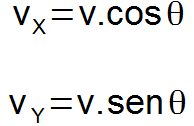In the definitions above, θ is the angle formed between the velocity vector and the horizontal.

Vertical movement

The movement performed by the body in the vertical is under the influence of the acceleration of gravity . Thus, it can be classified as uniformly varied rectilinear motion . From Torricelli’s equation , it is possible to determine the maximum height reached by the obliquely thrown object.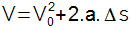Applying the above equation to the oblique throw, we can write that: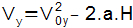The negative sign of the above equation is due to the fact that the motion is upwards and the gravity acceleration vector points vertically downwards. The difference in direction between displacement and acceleration causes the sign of this quantity to be negative. The height (H) corresponds to the displacement (ΔS) and the velocities considered are the initial and final components of the velocity vector on the y axis. Thus, we can determine the maximum height of an object during an oblique launch as follows: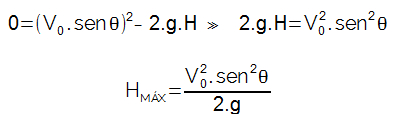horizontal movement

Horizontally, the body is not influenced by acceleration, so the movement is classified as rectilinear and uniform . From the position time function for uniform rectilinear motion , we can define the horizontal range of the object.

Note that the difference between the final position (s) and the initial position (s 0 ) was called A and represents the horizontal reach of a body in an oblique throw. The time considered should be the total time taken for the object to reach the maximum height and return to the ground. In the study of vertical launch ( free fall ), the time of ascent (t S ) to the maximum height for an object in upward motion is given by the ratio of the velocity on the y axis to the acceleration of gravity. Therefore, we can write: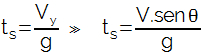Once the object returns to the ground, the value to be considered is twice the ascent time. So, we can write: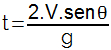Applying the definition of total time to the maximum range equation, we have:The product 2.cosθ.senθ has the term sin2θ as its trigonometric identity . Now we can finally define the maximum range of a body: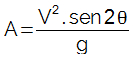launch angle

The maximum range acquired by a body, as a function of its initial velocity and the acceleration due to gravity, is determined when the value assigned to sin2θ is the greatest possible. The maximum sine value is 1 and corresponds to an angle of 90°. Therefore, when the launch angle is 45°, the sine value counted is the sine of 90° (sin2.45º = sin90º = 1), and the range is the maximum possible.

the horizontal ranges referring to different initial launch angles. In the long jump, shot put, hammer throw and javelin throw, the athlete’s objective is to achieve the greatest possible horizontal distance. Athletes train so that the throwing angle of the objects is as close as possible to 45° so that the range of the thrown object is as much as possible.

Check Also
Close